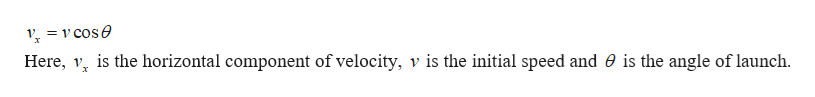A stone is thrown with an initial speed of 15 m/s at an angle of 53 ° above the horizontal from the top of a 35 m building. If g = 9.8 m/s 2 and air resistance is negligible, then what is the magnitude of the horizontal component of velocity as the rock strikes the ground? a.7.5 m/s b.9.0 m/s c.29 m/s d.12 m/s

Question

A stone is thrown with an initial speed of 15 m/s at an angle of 53 ° above the horizontal from the top of a 35 m building. If g = 9.8 m/s 2 and air resistance is negligible, then what is the magnitude of the horizontal component of velocity as the rock strikes the ground?

a.
7.5 m/s

b.
9.0 m/s

c.
29 m/s

d.
12 m/s

Step 1

The stone is in projectile motion. The stone has no acceleration in the horizontal direction since it is falling under gravity alone which acts in vertical direction. Since the stone has no acceleration in horizontal direction, its horizontal component of velocity will be the same throughout the motion or the velocity of the stone in horizontal direction as it strikes the ground will be equal to its initial horizontal velocity. The equation for the horizontal velocity of a projectile is given byhelp_outlineImage TranscriptioncloseV =cose Here, v is the horizontal component of velocity, v is the initial speed and 0 is the angle of launch fullscreen
Step 2

Substitute the numerical values...

Want to see the full answer?

See Solution

Want to see this answer and more?

Our solutions are written by experts, many with advanced degrees, and available 24/7

See Solution
Tagged in

Newtons Laws of Motion